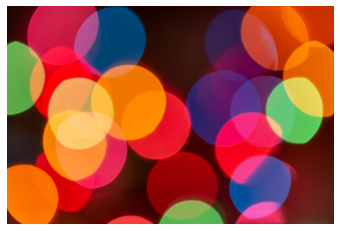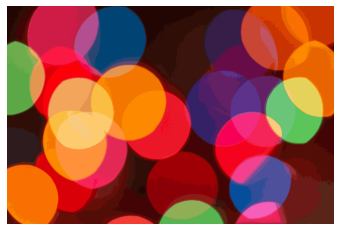Open in App
Not now

# Color Quantization using K-Means in Scikit Learn

• Difficulty Level : Hard
• Last Updated : 18 Feb, 2023

In this article, we shall play around with pixel intensity value using Machine Learning Algorithms. The goal is to perform a Color Quantization example using KMeans in the Scikit Learn library

### Color Quantization

Color Quantization is a technique in which the color spaces in an image are reduced to the number of distinct colors. Even after the color quantization, the new image is visually similar to the original image.

Color quantization is majorly used for displaying or visualizing images with many color spaces on those devices that can only display a limited number of colors, mainly due to memory limitations. This technique enables compression algorithms to render an image in those devices where the display is restricted to a number of shades.

K-Means is an Unsupervised Learning Clustering Algorithm that deals with density-based clustering. In this article, we shall use the K-Means algorithm to perform color quantization in an Image.

### Color Quantization using K-Means in Scikit Learn

Firstly, we will import the necessary modules:

## Python3

 `import` `numpy as np``import` `cv2``import` `matplotlib.pyplot as plt``from` `sklearn.cluster ``import` `KMeans`

If the above code gives you ImportError, then use the pip install command to install the packages.

Then, we need to read the Image for that we will use OpenCV.

## Python3

 `original_image ``=` `cv2.imread(``"lena.jpg"``)``plt.imshow(cv2.cvtColor(original_image, cv2.COLOR_BGR2RGB))``plt.axis(``"off"``)`

Output:After that we need to reshape the image i.e. flatten the image, to feed in model.

## Python3

 `reshaped_image ``=` `np.reshape(``    ``original_image,``      ``((original_image.shape[``0``] ``*` `original_image.shape[``1``]), ``3``))`

KMeans is a clustering algorithm, the k value follows a procedure. The procedure consists of applying the KMeans algorithm with a number of clusters that is equal to the number of colors you want to perform the quantization operation.

## Python3

 `model ``=` `KMeans(n_clusters``=``64``)``target ``=` `model.fit_predict(reshaped_image)``color_space ``=` `model.cluster_centers_`

Since the obtained color_space is in float, we need to convert it into an unsigned integer to visualize the image. We reshape back the original image shape i.e., to undo flattening.

## Python3

 `output_image ``=` `np.reshape(color_space.astype(``    ``"uint8"``)[target], (original_image.shape[``0``], original_image.shape[``1``], ``3``))``con_image ``=` `cv2.cvtColor(output_image, cv2.COLOR_BGR2RGB)``plt.imshow(con_image)``plt.axis(``"off"``)`

Output:### Conclusion

The images looks similar but the output image is much compressible as compared to the input image. We can use different n_clusters values for better results.

My Personal Notes arrow_drop_up